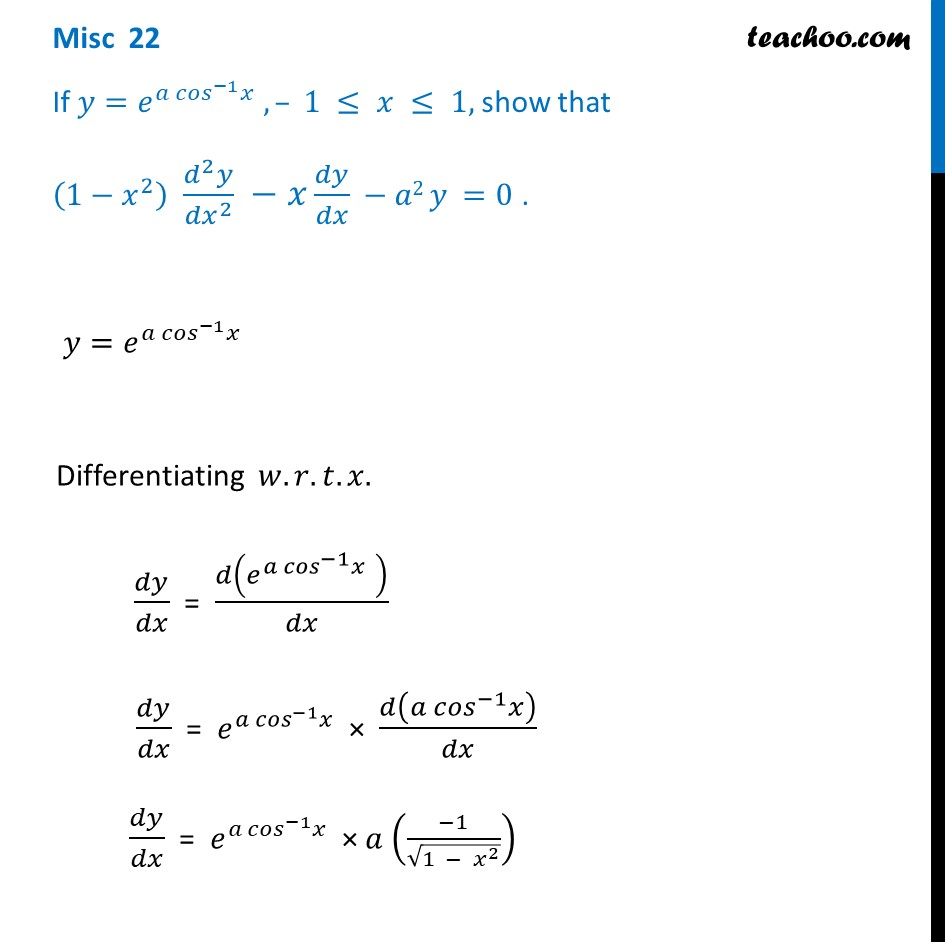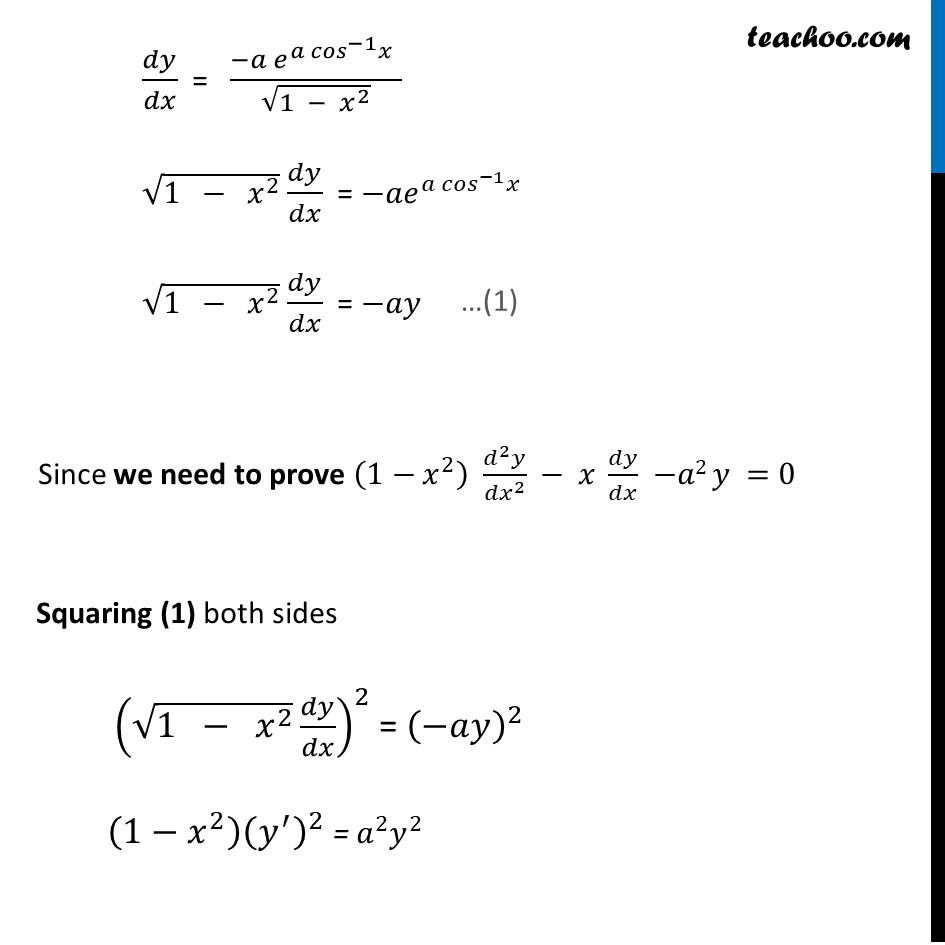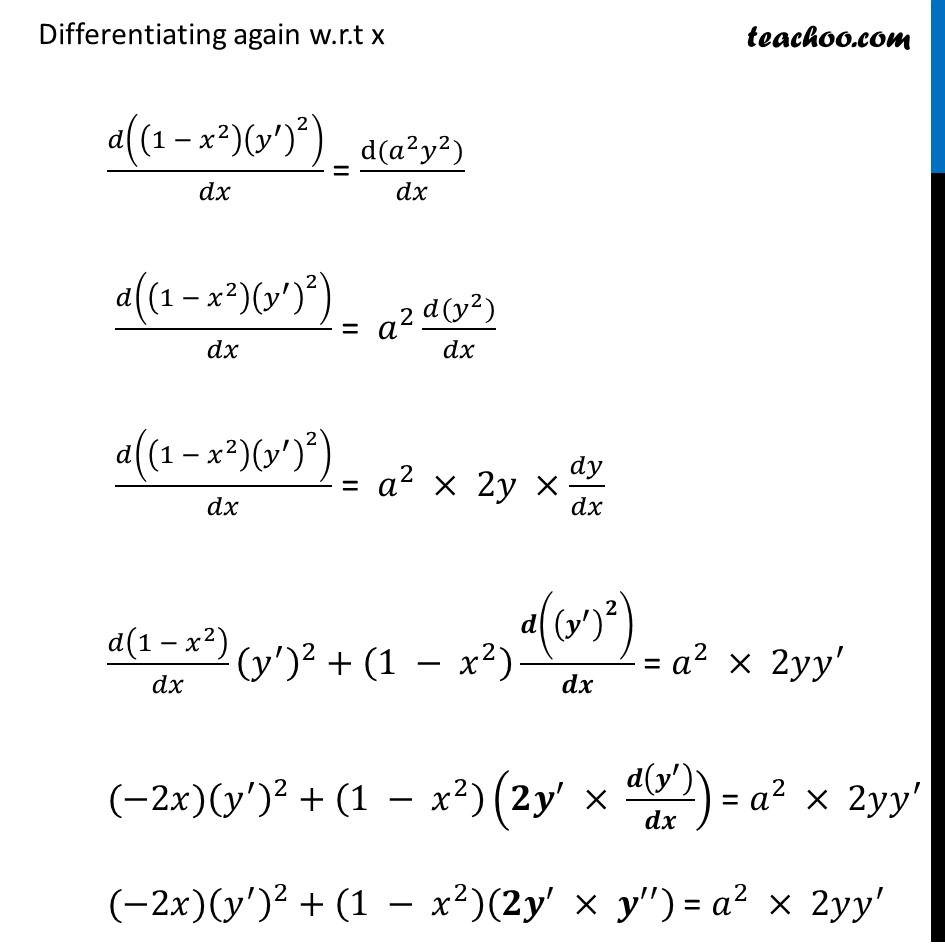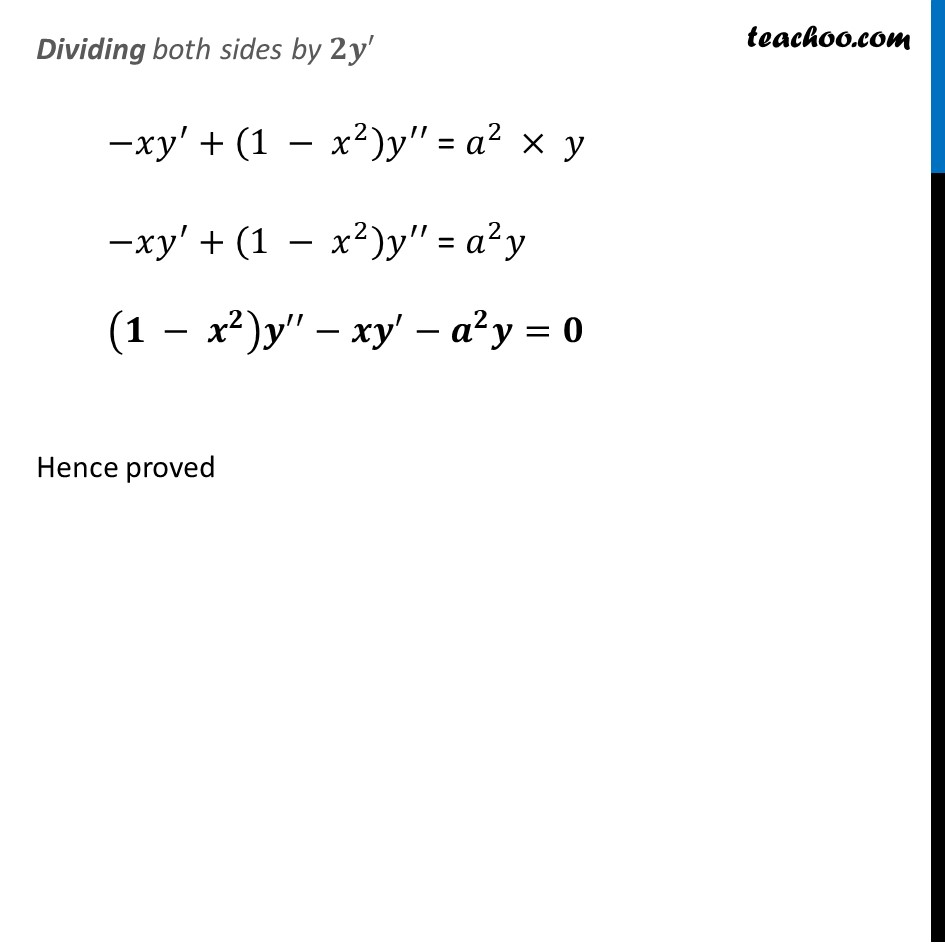Logarithmic Differentiation - Type 1

Chapter 5 Class 12 Continuity and Differentiability
Concept wiseLearn in your speed, with individual attention - Teachoo Maths 1-on-1 Class

### Transcript

Misc 23 If 𝑦=𝑒^(〖𝑎 𝑐𝑜𝑠〗^(−1) 𝑥) , – 1 ≤ 𝑥 ≤ 1, show that (1−𝑥^2 ) (𝑑^2 𝑦)/〖𝑑𝑥〗^2 −𝑥 𝑑𝑦/𝑑𝑥 − 𝑎2 𝑦 =0 . 𝑦=𝑒^(〖𝑎 𝑐𝑜𝑠〗^(−1) 𝑥) Differentiating 𝑤.𝑟.𝑡.𝑥. 𝑑𝑦/𝑑𝑥 = 𝑑(𝑒^(〖𝑎 𝑐𝑜𝑠〗^(−1) 𝑥" " ) )/𝑑𝑥 𝑑𝑦/𝑑𝑥 = 𝑒^(〖𝑎 𝑐𝑜𝑠〗^(−1) 𝑥" " ) × 𝑑(〖𝑎 𝑐𝑜𝑠〗^(−1) 𝑥)/𝑑𝑥 𝑑𝑦/𝑑𝑥 = 𝑒^(〖𝑎 𝑐𝑜𝑠〗^(−1) 𝑥" " ) × 𝑎 ((−1)/√(1 − 𝑥^2 )) 𝑑𝑦/𝑑𝑥 = (−𝑎 𝑒^(〖𝑎 𝑐𝑜𝑠〗^(−1) 𝑥" " ))/√(1 − 𝑥^2 ) √(1 − 𝑥^2 ) 𝑑𝑦/𝑑𝑥 = −𝑎𝑒^(〖𝑎 𝑐𝑜𝑠〗^(−1) 𝑥" " ) √(1 − 𝑥^2 ) 𝑑𝑦/𝑑𝑥 = −𝑎𝑦 Since we need to prove (1−𝑥^2 ) (𝑑^2 𝑦)/〖𝑑𝑥〗^2 − 𝑥 𝑑𝑦/𝑑𝑥 −𝑎2 𝑦 =0 Squaring (1) both sides (√(1 − 𝑥^2 ) 𝑑𝑦/𝑑𝑥)^2 = (−𝑎𝑦)^2 (1−𝑥^2 ) (𝑦^′ )^2 = 𝑎^2 𝑦^2 Differentiating again w.r.t x 𝑑((1 − 𝑥^2 ) (𝑦^′ )^2 )/𝑑𝑥 = (d(𝑎^2 𝑦^2))/𝑑𝑥 𝑑((1 − 𝑥^2 ) (𝑦^′ )^2 )/𝑑𝑥 = 𝑎^2 (𝑑(𝑦^2))/𝑑𝑥 𝑑((1 − 𝑥^2 ) (𝑦^′ )^2 )/𝑑𝑥 = 𝑎^2 × 2𝑦 ×𝑑𝑦/𝑑𝑥 𝑑(1 − 𝑥^2 )/𝑑𝑥 (𝑦^′ )^2+(1 − 𝑥^2 ) 𝒅((𝒚^′ )^𝟐 )/𝒅𝒙 = 𝑎^2 × 2𝑦𝑦^′ (−2𝑥)(𝑦^′ )^2+(1 − 𝑥^2 )(𝟐𝒚^′ × 𝒅(𝒚^′ )/𝒅𝒙) = 𝑎^2 × 2𝑦𝑦^′ (−2𝑥)(𝑦^′ )^2+(1 − 𝑥^2 )(𝟐𝒚^′ × 𝒚^′′ ) = 𝑎^2 × 2𝑦𝑦^′ Dividing both sides by 𝟐𝒚^′ −𝑥𝑦^′+(1 − 𝑥^2 ) 𝑦^′′ = 𝑎^2 × 𝑦 −𝑥𝑦^′+(1 − 𝑥^2 ) 𝑦^′′ = 𝑎^2 𝑦 (𝟏 − 𝒙^𝟐 ) 𝒚^′′−𝒙𝒚^′−𝒂^𝟐 𝒚=𝟎 Hence proved